Notebook

# Factor Combination Theory and Tools¶

The purpose of this notebook is to provide a set of functions to allow the user to explore how a combination of uncorrelated and possibly "interacting" factors can result in an enhanced signal. It also provides some tools to detect interaction effects between factors. <br><br>

Note: The following link does a good job of illustrating how to interpret factor interaction plots (although it is in a completely different context from the finance field). https://courses.washington.edu/smartpsy/interactions.htm

In :
import pandas as pd
import numpy as np
import matplotlib.pyplot as plt
import seaborn as sns
import matplotlib.cm as cm

from scipy import stats
import alphalens as al


### Factor Interaction Analysis Functions¶

These will be used later when analyzing the combination of factors.

In :
def mean_return_by_quantile(factor_data,
by_date=False,
by_group=False,
demeaned=True,
factor_groupers=['factor_quantile']):
"""
Computes mean returns for factor quantiles across
provided forward returns columns.
Parameters
----------
factor_data : pd.DataFrame - MultiIndex
A MultiIndex DataFrame indexed by date (level 0) and asset (level 1),
containing the values for a single alpha factor, forward returns for
each period, the factor quantile/bin that factor value belongs to, and
(optionally) the group the asset belongs to.
- See full explanation in utils.get_clean_factor_and_forward_returns
by_date : bool
If True, compute quantile bucket returns separately for each date.
by_group : bool
If True, compute quantile bucket returns separately for each group.
demeaned : bool
Compute demeaned mean returns (long short portfolio)
Returns demeaning will occur on the group level.
factor_groupers: list
list of column names (strings) for the factor quantiles to group by
Returns
-------
mean_ret : pd.DataFrame
Mean period wise returns by specified factor quantile.
std_error_ret : pd.DataFrame
Standard error of returns by specified quantile.
"""

grouper = [factor_data.index.get_level_values('date')] + ['group']
factor_data = al.utils.demean_forward_returns(factor_data, grouper)
elif demeaned:
factor_data = al.utils.demean_forward_returns(factor_data)
else:
factor_data = factor_data.copy()

grouper = factor_groupers
if by_date:
grouper.append(factor_data.index.get_level_values('date'))

if by_group:
grouper.append('group')

group_stats = factor_data.groupby(grouper)[
al.utils.get_forward_returns_columns(factor_data.columns)] \
.agg(['mean', 'std', 'count'])

mean_ret = group_stats.T.xs('mean', level=1).T

std_error_ret = group_stats.T.xs('std', level=1).T \
/ np.sqrt(group_stats.T.xs('count', level=1).T)

return mean_ret, std_error_ret

def plot_multi_factor_quantile_returns(mean_ret_by_quantile, period, ax=None):
"""
Plots mean period wise returns for factor quantiles.
Parameters
----------
mean_ret_by_q : pd.DataFrame
DataFrame with quantiles, (group) and mean period wise return values.
period: pandas.Timedelta or string
Length of period for which the returns are computed (e.g. 1 day)
if 'period' is a string it must follow pandas.Timedelta constructor
format (e.g. '1 days', '1D', '30m', '3h', '1D1h', etc)
ax : matplotlib.Axes, optional
Axes upon which to plot.
Returns
-------
ax : matplotlib.Axes
"""
if ax is None:
fig, ax = plt.subplots(figsize=(6, 6))

sns.heatmap(mean_ret_by_quantile[period].unstack(), annot=True,
cmap=cm.coolwarm_r, ax=ax, center=0)
ax.set(title="Mean {} Returns".format(period))
else:
sns.heatmap(mean_ret_by_quantile[period].unstack(), annot=True,
cmap=cm.coolwarm_r, ax=ax, center=0)
ax.set(title="Mean {} Returns".format(period))


## Generate Simulated Factor Values and Returns¶

Let's generate some randomly uncorrelated factor values in addition to a return stream that is a function of the factor values and their interaction.

$r = \beta_1f_1 + \beta_2f_2 + \beta_3f_1f_2 + \epsilon$

In :
def generate_factor(n_stocks, n_periods):
"""Generate random factor values for given number of stocks and periods

Parameters
-----------
n_stocks: int
Number of stocks in simulation
n_periods: int
Number of days

Return
-------
pd.Series
Multi-index series of factor values (index by date, then asset)
"""
factor = np.random.normal(0, size=n_stocks * n_periods)
date_idx = pd.DatetimeIndex(start='2003-01-01', periods=n_periods, freq='B')
idx = pd.MultiIndex.from_product([date_idx, (range(n_stocks))])
factor = pd.Series(factor, idx)
factor.index.names=['date', 'asset']
return factor

def generate_simulated_returns(factor_1, factor_2, factor_1_coef, factor_2_coef, interaction_coef):
"""Generate simulated returns as a function of the factor_1, factor_2, and factor_1*factor_2
values.

Parameters
----------
factor_1, factor_2: pd.Series
Series indexed by date, then asset containing factor values
factor_1_coef, factor_2_coef, interaction_coef: float

Returns
-------
pd.Series:
Daily return series indexed by date and then asset
"""
ret = (factor_1_coef * factor_1) + (factor_2_coef * factor_2) + \
(interaction_coef * factor_1*factor_2)
noise = np.random.normal(0,0.02, size=len(factor_1))
ret = ret + noise
return pd.Series(ret, index=factor_1.index)


### The following function will be used at the end of the notebook to quickly generate additional examples¶

In :
def simulate_and_plot_results(n_stocks, n_periods, factor_1_coef, factor_2_coef, interaction_coef):
"""Perform Entire Simulation and Plot Results in One Step"""
factor_1 = generate_factor(N_STOCKS, N_PERIODS)
factor_2 = generate_factor(N_STOCKS, N_PERIODS)

sim_returns = generate_simulated_returns(factor_1, factor_2, factor_1_coef, factor_2_coef, interaction_coef)

factor_data_1 = al.utils.get_clean_factor(factor_1, pd.DataFrame({'1D': sim_returns}))
factor_data_2 = al.utils.get_clean_factor(factor_2, pd.DataFrame({'1D': sim_returns}))
factor_data_1.rename(columns={'factor': 'factor_1', 'factor_quantile': 'factor_1_quantile'}, inplace=True)
factor_data_2.rename(columns={'factor': 'factor_2', 'factor_quantile': 'factor_2_quantile'}, inplace=True)
multi_factor_data = factor_data_1.join(factor_data_2[['factor_2', 'factor_2_quantile']])

mean_ret_by_q = mean_return_by_quantile(multi_factor_data,
factor_groupers=['factor_1_quantile','factor_2_quantile'])
print "------------------------------------------------------------"
print  "Mean Return by Factor Quantile for Each Factor Individually"
print  "-----------------------------------------------------------"
print mean_ret_by_q.groupby(level=0).mean(), '\n', mean_ret_by_q.groupby(level=1).mean()
print "------------------------------------------------------------"
print "Mean 1 Day Returns for Each Factor 1 and 2 Quantile Intersection"
print "----------------------------------------------------------------"
plot_multi_factor_quantile_returns(mean_ret_by_q, '1D', ax=None)
mean_ret_by_q['1D'].unstack().plot(title='Factor Interaction Plot')
plt.gca().set_ylabel('Return');



### Set Parameters for the Simulation and Generate Data¶

Let's choose some parameters for our simulation and then generate the simulated factor values and stock returns. For this simulation, I am going to set the factor_2 coefficient to 0. In other words, the value of factor 2 will not be predictive of future returns. However, the interaction coefficient will have a positive loading.

In :
N_STOCKS = 5
N_PERIODS = 1000
FACTOR_1_COEF = 0.05
FACTOR_2_COEF = 0
INTERACTION_COEF = 0.05

factor_1 = generate_factor(N_STOCKS, N_PERIODS)
factor_2 = generate_factor(N_STOCKS, N_PERIODS)

sim_returns = generate_simulated_returns(factor_1, factor_2, FACTOR_1_COEF, FACTOR_2_COEF, INTERACTION_COEF)


### Distribution of Simulated Returns¶

This is simply exploratory, just to see what kind of return distribution was generated. There appears to be some positive excess kurtosis.

In :
fig, ax = plt.subplots(ncols=2)
ax.hist(sim_returns, bins=30);
ax.set(title='Distribution of Simulated Returns')
stats.probplot(sim_returns, plot=ax)
stats.describe(sim_returns)

Out:
DescribeResult(nobs=5000, minmax=(-0.479193910090562, 0.43861417253908219), mean=-0.00073856949450228125, variance=0.0056630401883038008, skewness=-0.03691830849911114, kurtosis=3.377571351421362)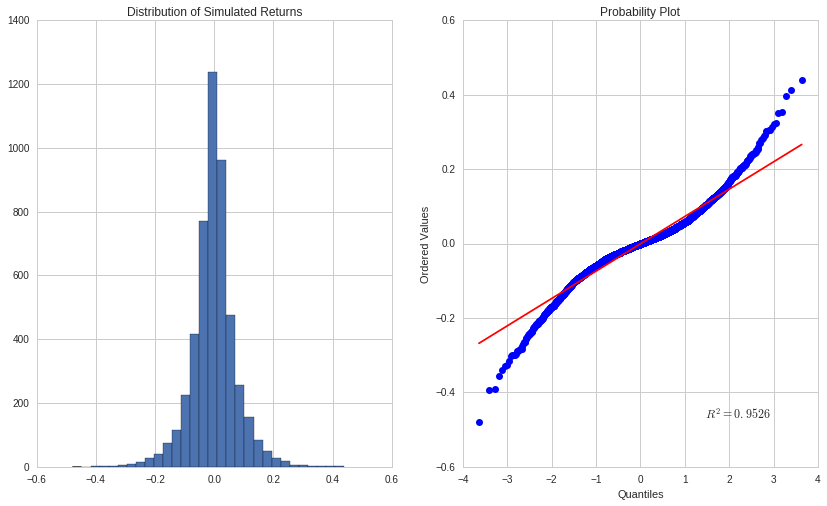### Evaluate Correlation between Factor Values¶

Let's verify that the correlation between factors is zero.

In :
h = sns.jointplot(factor_1, factor_2, annot_kws={'title': 'Factor Correlation'});
h.set_axis_labels('factor_1', 'factor_2', fontsize=16);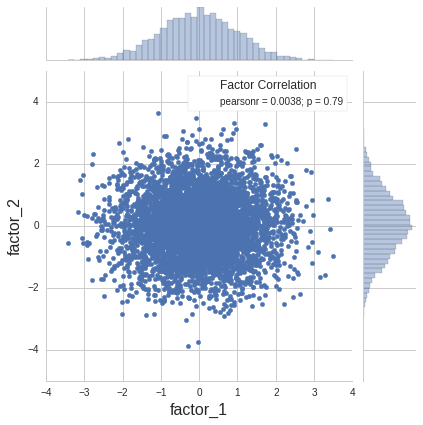### Plot Factor Values vs. Returns¶

As opposed to generating alphalens output for these, I just decided to show a simple scatter plot for each factor and factor interaction to show whether each factor (or interaction term) was predictive of future returns on its own.

In :
fig, axes = plt.subplots(nrows=2, ncols=2)
for factor, ax, title in zip([factor_1, factor_2, factor_1*factor_2] ,axes.flat,
['Factor 1', 'Factor 2', 'Interaction']):
sns.regplot(factor, sim_returns, ax=ax)
ax.set(title=title, xlabel='Factor Value', ylabel='Return')
fig.tight_layout()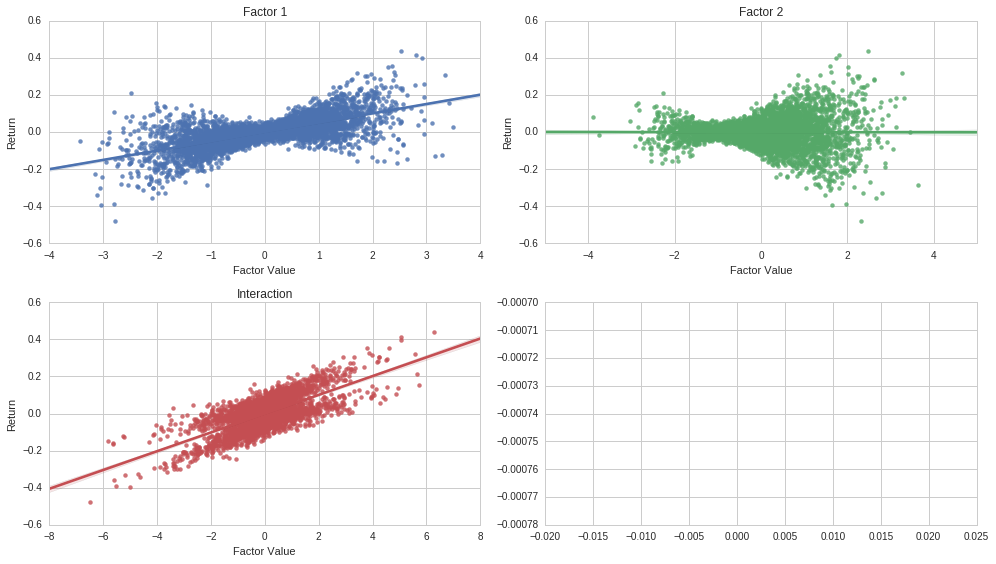### Generate Data Structure to Feed into Interaction Analysis Functions Created Above¶

In :
factor_data_1 = al.utils.get_clean_factor(factor_1, pd.DataFrame({'1D': sim_returns}))
factor_data_2 = al.utils.get_clean_factor(factor_2, pd.DataFrame({'1D': sim_returns}))
factor_data_1.rename(columns={'factor': 'factor_1', 'factor_quantile': 'factor_1_quantile'}, inplace=True)
factor_data_2.rename(columns={'factor': 'factor_2', 'factor_quantile': 'factor_2_quantile'}, inplace=True)
multi_factor_data = factor_data_1.join(factor_data_2[['factor_2', 'factor_2_quantile']])

Dropped 0.0% entries from factor data: 0.0% in forward returns computation and 0.0% in binning phase (set max_loss=0 to see potentially suppressed Exceptions).
max_loss is 35.0%, not exceeded: OK!
Dropped 0.0% entries from factor data: 0.0% in forward returns computation and 0.0% in binning phase (set max_loss=0 to see potentially suppressed Exceptions).
max_loss is 35.0%, not exceeded: OK!

Out:
1D factor_1 factor_1_quantile factor_2 factor_2_quantile
date asset
2003-01-01 0 0.154096 1.479668 5 0.941797 4
1 0.069903 0.993258 3 -0.385766 2
2 -0.010461 -0.371420 1 0.594015 3
3 -0.079000 0.995555 4 -1.760521 1
4 0.024042 0.202703 2 1.975039 5
In :
mean_ret_by_q = mean_return_by_quantile(multi_factor_data,
factor_groupers=['factor_1_quantile','factor_2_quantile'])

In :
print  "Mean Return by Factor Quantile for Each Factor Individually"
print  "-----------------------------------------------------------"
print mean_ret_by_q.groupby(level=0).mean(), '\n', mean_ret_by_q.groupby(level=1).mean()

Mean Return by Factor Quantile for Each Factor Individually
-----------------------------------------------------------
1D
factor_1_quantile
1                 -0.061164
2                 -0.024412
3                 -0.000660
4                  0.025489
5                  0.058594
1D
factor_2_quantile
1                 -0.000674
2                 -0.000961
3                  0.000577
4                 -0.002400
5                  0.001305

In :
print "Mean 1 Day Returns for Each Factor 1 and 2 Quantile Intersection"
print "----------------------------------------------------------------"
plot_multi_factor_quantile_returns(mean_ret_by_q, '1D', ax=None)

Mean 1 Day Returns for Each Factor 1 and 2 Quantile Intersection
----------------------------------------------------------------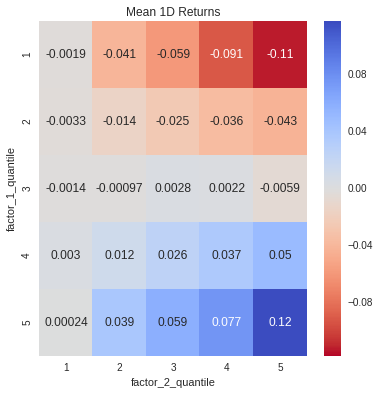### Factor Interaction Plot¶

This plot illustrates the heat map above in a different type of visualization. The key in this plot is to look at how the slope of the line changes as we alter the factor_2_quantile variable. Since the slope changes as we change the factor_2_quantile, it suggests that there is an "non-additive" interaction between factor 1 and factor 2. In fact, this is a case where factor 2 had no predictive ability by itself. However, when combined with factor 1 it actually can enhance the predictability of the entire model.

In :
mean_ret_by_q['1D'].unstack().plot(title='Factor Interaction Plot');
plt.ylabel('Return');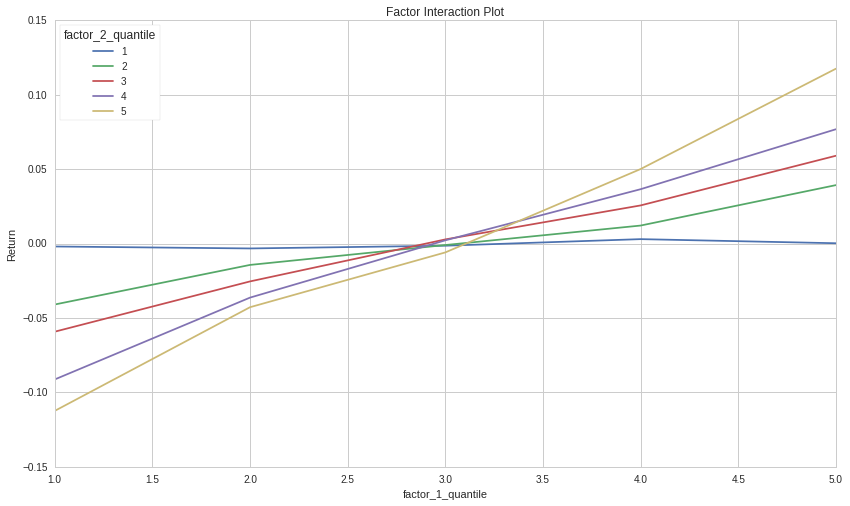Note: If it was desirable to elminate the individual factor exposure and only have exposure to the "interaction factor", it might make sense to neutralize your factor exposure by going long the (Q5, Q5) and (Q1, Q1) bins while going short the (Q1, Q5) and (Q5, Q1) bins.

## Example 2: Uncorrelated Factors with No Interaction¶

In :
N_STOCKS = 5
N_PERIODS = 1000
FACTOR_1_COEF = 0.05
FACTOR_2_COEF = 0.05
INTERACTION_COEF = 0.

simulate_and_plot_results(N_STOCKS, N_PERIODS, FACTOR_1_COEF, FACTOR_2_COEF, INTERACTION_COEF)

Dropped 0.0% entries from factor data: 0.0% in forward returns computation and 0.0% in binning phase (set max_loss=0 to see potentially suppressed Exceptions).
max_loss is 35.0%, not exceeded: OK!
Dropped 0.0% entries from factor data: 0.0% in forward returns computation and 0.0% in binning phase (set max_loss=0 to see potentially suppressed Exceptions).
max_loss is 35.0%, not exceeded: OK!
------------------------------------------------------------
Mean Return by Factor Quantile for Each Factor Individually
-----------------------------------------------------------
1D
factor_1_quantile
1                 -0.059193
2                 -0.023659
3                  0.000865
4                  0.023500
5                  0.058474
1D
factor_2_quantile
1                 -0.059858
2                 -0.025026
3                 -0.001189
4                  0.026909
5                  0.059151
------------------------------------------------------------
Mean 1 Day Returns for Each Factor 1 and 2 Quantile Intersection
----------------------------------------------------------------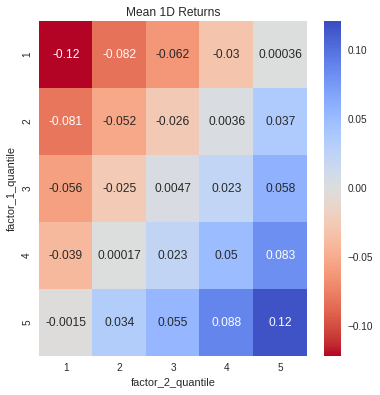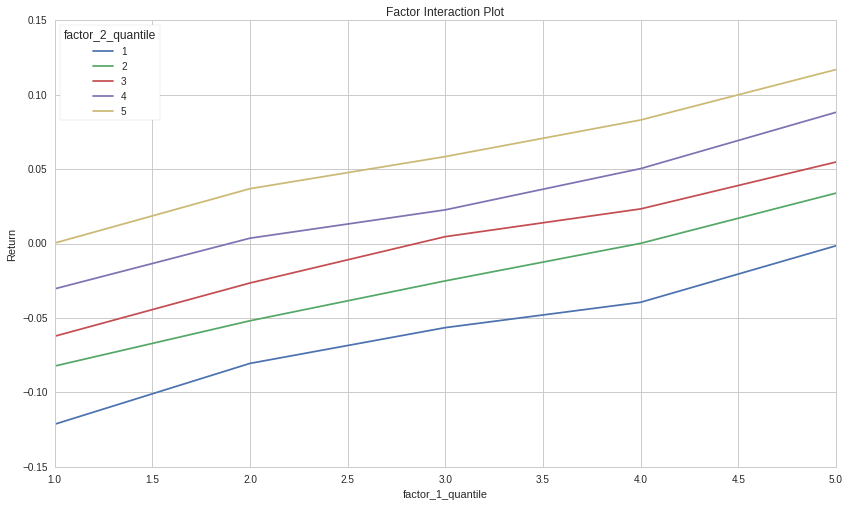Note how both factors seem to have an effect on return, but the effect is "additive". There is no change in slope when varying the factor_2_quantile.

### Example 3: Both Factors Predicitve with an Interaction Term¶

In :
N_STOCKS = 5
N_PERIODS = 1000
FACTOR_1_COEF = 0.05
FACTOR_2_COEF = 0.05
INTERACTION_COEF = 0.05

simulate_and_plot_results(N_STOCKS, N_PERIODS, FACTOR_1_COEF, FACTOR_2_COEF, INTERACTION_COEF)

Dropped 0.0% entries from factor data: 0.0% in forward returns computation and 0.0% in binning phase (set max_loss=0 to see potentially suppressed Exceptions).
max_loss is 35.0%, not exceeded: OK!
Dropped 0.0% entries from factor data: 0.0% in forward returns computation and 0.0% in binning phase (set max_loss=0 to see potentially suppressed Exceptions).
max_loss is 35.0%, not exceeded: OK!
------------------------------------------------------------
Mean Return by Factor Quantile for Each Factor Individually
-----------------------------------------------------------
1D
factor_1_quantile
1                 -0.056899
2                 -0.024452
3                 -0.000953
4                  0.027184
5                  0.054621
1D
factor_2_quantile
1                 -0.056111
2                 -0.025018
3                 -0.000684
4                  0.021922
5                  0.059389
------------------------------------------------------------
Mean 1 Day Returns for Each Factor 1 and 2 Quantile Intersection
----------------------------------------------------------------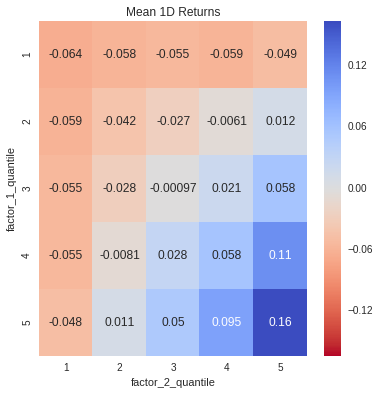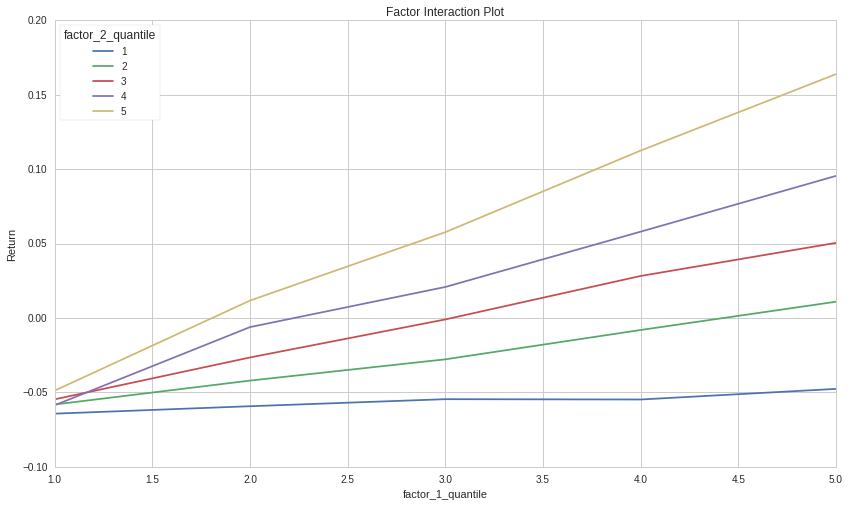The individual positive effect for both variables and interaction effect is clearly visible.

### Next Steps¶

1. It may be educational to modify the factor generation function to allow for factor values that are correlated.
2. Use an example of two real-life factors, and analyze potential combination and interaction effects.
3. Suggest new standard charts/tools for analyzing combinations of factors that could be used in an alphalens factor combination/interaction tearsheet.